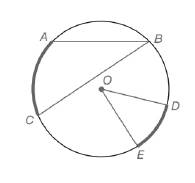Chapter 6.1, Problem 5EElementary Geometry For College St...

7th Edition
Alexander + 2 others
ISBN: 9781337614085

Solutions

Chapter
SectionElementary Geometry For College St...

7th Edition
Alexander + 2 others
ISBN: 9781337614085
Textbook Problem

For Exercises 1 to 8, use the figure provided.Exercises 1-8If m ∠ B = 28.3 ∘ ,  find m A C ⌢ .

To determine

To calculate:

The mAC, mB=28.3o by using the provided figure.

Explanation

Given:

The provided figure is given below:

Theorem used:

The measure of an inscribed angle of a circle is one-half the measure of its intercepted arc.

Calculation:

Given, mB=28.3o

Since, the measure of an inscribed angle of a circle is one-half the measure of its intercepted arc.

mB=12mAC28

Still sussing out bartleby?

Check out a sample textbook solution.

See a sample solution

The Solution to Your Study Problems

Bartleby provides explanations to thousands of textbook problems written by our experts, many with advanced degrees!

Get Started

Prove, using Definition 9, that limxx3=. Definition 9

Single Variable Calculus: Early Transcendentals

For , which vector, a, b, c, or d, best represents F(1, 1)? a b c d

Study Guide for Stewart's Multivariable Calculus, 8th

What is the partial fraction form of ?

Study Guide for Stewart's Single Variable Calculus: Early Transcendentals, 8th